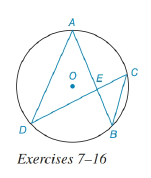Chapter 6.3, Problem 13EElementary Geometry For College St...

7th Edition
Alexander + 2 others
ISBN: 9781337614085

Solutions

Chapter
SectionElementary Geometry For College St...

7th Edition
Alexander + 2 others
ISBN: 9781337614085
Textbook Problem

In the figure for Exercises 7 to 16, O is the center of the circle. See Theorem 6.3.5.Given: A E = x 2 , E B = 12 , D E = x + 6 3 ,     a n d     E C = 9 Find: x and AE.To determine

To find:

To find x and AE.

Explanation

Given that, AE=x2,EB=12,DE=x+63,andEC=9

The diagrammatic representation is given below,

Theorem:

If two chords intersect within a circle, then the product of the lengths of the segments (parts) of one chord is equal to the product of the lengths of the segments of the other chord.

By using the theorem to get the following,

AEEB=DEEC

Substitute the values AE=x2,EB=12,DE=x+63,andEC=9 in the above equation to get the following,

Still sussing out bartleby?

Check out a sample textbook solution.

See a sample solution

The Solution to Your Study Problems

Bartleby provides explanations to thousands of textbook problems written by our experts, many with advanced degrees!

Get Started

In problems 1-6, perform the indicated matrix operations with the following matrices.

Mathematical Applications for the Management, Life, and Social Sciences

True or False: f(x) = 4x2 8x + 1 is increasing on (0, 1).

Study Guide for Stewart's Single Variable Calculus: Early Transcendentals, 8th

It does not exist.

Study Guide for Stewart's Multivariable Calculus, 8th